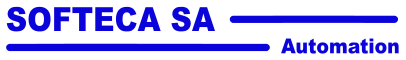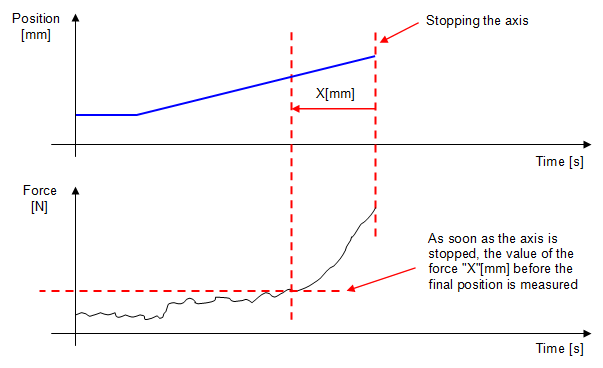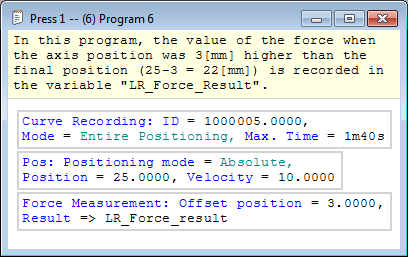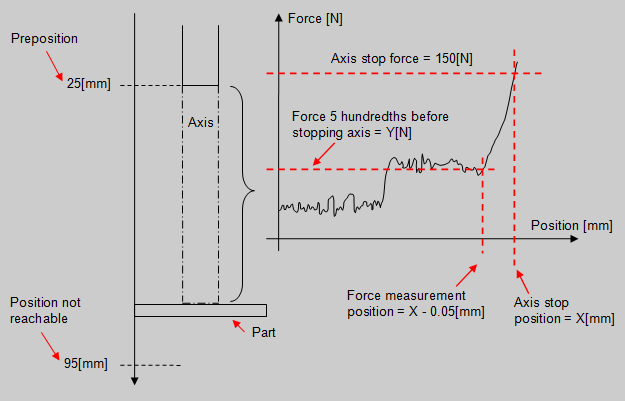# Presse universelle MecaMotion parNavigation: Part program instructions > Controls "Post-process force measurement" control instruction

"Post-process force measurement" control instruction

This instruction allows the value of the force to be recorded in a "result" variable "X"[mm] before the end of a positioning.Figure 1: Schematic explanation of the instruction Post-Process force measurement

This instruction must be used in conjunction with the curve recording instruction. Indeed, the value of the force "X"[mm] before the final position will be searched in the registration table.

This "post-process" instruction, unlike other controls, comes after the positioning in which the measurement is to be performed.Figure 2: Example explaining how to use the "Post-process force measurement" control

Below is a list of the instruction's input and output parameters:

Parameter name

Declaration

Type of data

Default value

Description

Position offset measurement [mm]

Input

LREAL

0[mm]

Distance before the final position where the force must be recorded

Result [N]

Output

REAL

--

Measured force X[mm] before the end position

Table 1: List of instruction parameters

 Example of use: We want to know the force exerted on a part, 0.05 millimeter before the final force of 150[N] is reached. To do this, in the program in figure 4, we make a movement to the pre-position, then a movement with a stop on signal control if the force is greater than 150[N] and when this force is reached we stop the axis. Then, the instruction "post-process force measurement" will read from the registration table the force exerted on the part 0.05 millimeter before the final force has been detected (axis stop).Figure 3: Graphical explanation of the exampleFigure 4: Part program to measure the force 0.05[mm] before the final position